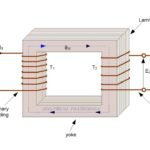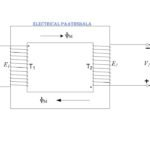# Ampere turns relation

In this blog we will discuss Ampere-turns relation. After going through this topic we will get an insight of how the mutual flux sets-up in the core of a transformer and also we will see why the mutual flux ɸM remains almost constant? We will also learn why transformer is a constant frequency device? So, here we go.

At all ordinary loads, the e.m.f. E1 induced in the primary by the mutual flux ɸM, is nearly equal to the primary terminal voltage V1 in magnitude. The e.m.f. E1 differs from primary terminal voltage V1 only by a small magnitude due to the small impedance drop (I1Z1) in the primary winding. Hence, E1 and V1 are nearly equal. Also, since V1 is constant, the induced e.m.f. must also be nearly constant.

Applying KVL in primary winding

V1 – I1Z1 – E1 = 0

∵ I1Z1 is a small impedance drop.

$${ V }_{ 1 }$$$$\approx$$$${ I }_{ 1 }$$

Now, since E1 is nearly constant the mutual flux ɸM also must be nearly constant at all normal loads and therefore the m.m.f. (N1I1) producing mutual flux ɸM as well as the iron losses must be nearly constant. Thus the exciting current Io must be constant at all normal loads on the transformer. Also Io is small in magnitude, generally being 2 to 6% of the rated primary current.

The exciting current is of small magnitude and generally differs considerably in phase from the total primary current. Therefore, it is normally neglected in comparison with the total primary current.

Now, if secondary is loaded then current I2 starts flowing in the secondary and due to this current I2 demagnetizing flux ɸ2 is produced by the secondary ampere-turns (N2I2) and this flux ɸ2 opposes the mutual flux ɸM according to the Lenz’s law. Due to the opposition offered by demagnetizing flux ɸ2 the main flux ɸM starts decreasing which results in decrease of the induced e.m.f. E1.

But as we know that e.m.f. E1 is almost constant and to keep this constant, the mutual flux ɸM must be restored to the original value. To achieve this, the primary winding draws an additional current I1 from the a.c. mains, such that the primary ampere turns (N1I1) is equal to the secondary ampere-turns (N2I2).

N1I1 = N2I2

∵$$\frac { I_{ 1 } }{ I_{ 2 } } =\frac { N_{ 2 } }{ N_{ 1 } }$$

The above equations are known as “Ampere turns Relation”.

If the transformer losses be neglected and unity power factor be assumed, then

$$\frac { E_{ 1 } }{ E_{ 2 } } =\frac { V_{ 1 } }{ V_{ 2 } } =\frac { N_{ 1 } }{ N_{ 2 } } =\frac { I_{ 2 } }{ I_{ 1 } }$$

i.e.   $$V_{ 1 }I_{ 1 }=V_{ 2 }I_{ 2 }$$

## Explanation:-

Referring to the figure given below, V1 is the supply voltage source connected to the primary winding of the transformer. I1 current flows through primary winding and due to magnetizing ampere-turns (N1I1) mutual flux ɸM sets up in the core of the transformer which in turns induce e.m.f. E1 in primary winding.

Now, let the secondary terminal be loaded and the load current be I2. Due to secondary ampere-turns (N2I2) a flux ɸ2 is produced and according to Lenz’s law, this flux ɸ2 oppose the main flux ɸM. This results in decrease of the main flux ɸM and hence primary induced e.m.f. E1 also decreases.

As we know that primary terminal voltage V1 and induced e.m.f. E1 is almost equal. But due to decrease in E1 a potential difference between V1 and E1 occurs which results in drawing of additional current I1through primary winding and this current I1produces an additional flux ɸ1by primary ampere-turns (N1I1’) in same direction of the main flux. This additional flux ɸ1 is equal and opposite to the flux ɸ2. Hence flux ɸ1 compensate the flux ɸ2 and keep the main flux ɸM constant.

The additional current I1in primary winding is given by

$$I_{ 1 }^{ ‘ }=(V_{ 1 }-E_{ 1 })/Z_{ 1 }$$

## Why the mutual flux (ɸM) of a transformer remains constant ?

As shown in the figure three fluxes (ɸM 1 and ɸ2) are circulating in the core of transformer in which ɸM and ɸ1 are circulating in the same direction and ɸ2 is circulating opposite direction. As flux ɸ1 and ɸ2 are equal and opposite they cancel out each other and that’s why the main or mutual flux ɸM of the transformer remains almost constant.

## Why Transformer is a constant frequency device ?

As the supply frequency to the transformer causes production of alternating flux ɸM in the core. So, the frequency of supply and flux ɸM is same. This flux ɸM links both primary as well as secondary winding which induce e.m.f. E1 and E2 in the primary and secondary windings respectively.

As e.m.f. E1 and E2 are induced by the same flux ɸM therefore the frequency of primary and secondary induced e.m.f. (E1 and E2) is same as the frequency of flux ɸM. Hence the frequency of flux ɸM and mutually induced e.m.f. in the secondary winding (E2) remains same as that of primary winding supply.

The other reason for same frequency on both sides is that since transformer is a static device so there is no relative motion between primary and secondary windings therefore the frequency of the induced voltage in secondary is same as the frequency of the applied voltage to the primary.

Hence, we can say that transformer is a constant frequency device because whatever is the supply frequency at input of the transformer, same is the value of frequency at the output of the transformer.

If you have any doubt regarding ampere turns or any other topic then please drop a comment below. You can join us on our Youtube channel.